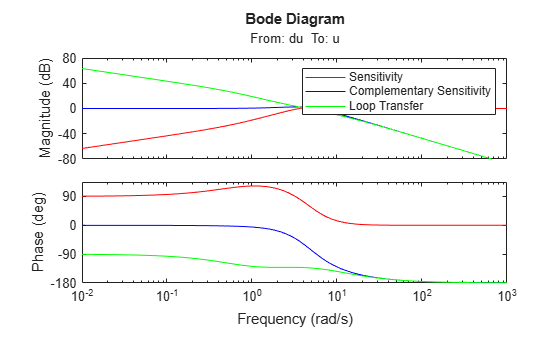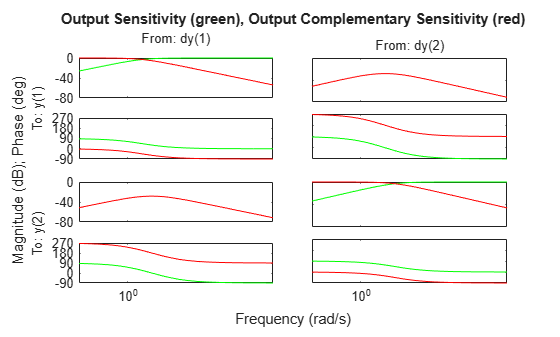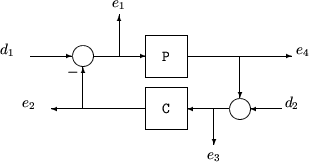# loopsens

Sensitivity functions of plant-controller feedback loop

## Syntax

``loops = loopsens(P,C)``

## Description

example

````loops = loopsens(P,C)` computes the multivariable sensitivity, complementary sensitivity, and open-loop transfer functions of the closed-loop system consisting of the controller `C` in negative feedback with the plant `P`. To compute the sensitivity functions for the system with positive feedback, use `loopsens(P,-C)`.```

## Examples

collapse all

Consider PI controller for a dominantly first-order plant, with the closed-loop bandwidth of 2.5 rads/sec. Since the problem is SISO, all gains are the same at input and output.

```gamma = 2; tau = 1.5; taufast = 0.1; P = tf(gamma,[tau 1])*tf(1,[taufast 1]); tauclp = 0.4; xiclp = 0.8; wnclp = 1/(tauclp*xiclp); KP = (2*xiclp*wnclp*tau - 1)/gamma; KI = wnclp^2*tau/gamma; C = tf([KP KI],[1 0]);```

Form the closed-loop (and open-loop) systems with `loopsens`, and plot Bode plots of the sensitivity functions at the plant input.

```loops = loopsens(P,C); bode(loops.Si,'r',loops.Ti,'b',loops.Li,'g') legend('Sensitivity','Complementary Sensitivity','Loop Transfer')```Finally, compare the open-loop plant gain to the closed-loop value of `PSi`.

```bodemag(P,'r',loops.PSi,'b') legend('Plant','Sensitivity*Plant')```Consider an integral controller for a constant-gain, 2-input, 2-output plant. For purposes of illustration, the controller is designed via inversion, with different bandwidths in each rotated channel.

```P = ss([2 3;-1 1]); BW = diag([2 5]); [U,S,V] = svd(P.d); % get SVD of Plant Gain Csvd = V*inv(S)*BW*tf(1,[1 0])*U'; % inversion based on SVD loops = loopsens(P,Csvd); bode(loops.So,'g',loops.To,'r',logspace(-1,3,120)) title('Output Sensitivity (green), Output Complementary Sensitivity (red)');```## Input Arguments

collapse all

Plant, specified as a dynamic system model, control design block, or static gain matrix. `P` can be SISO or MIMO, as long as `P*C` has the same number of inputs and outputs.

`P` can be continuous time or discrete time. If `P` is a generalized model (such as `genss` or `uss`) then `loopsens` uses the current or nominal value of all control design blocks in `P`.

Controller, specified as a dynamic system model, control design block, or static gain matrix. The controller can be any of the model types that `P` can be, as long as `P*C` has the same number of inputs and outputs. `loopsens` computes the sensitivity functions assuming a negative-feedback closed-loop system. To compute the sensitivity functions for the system with positive feedback, use `loopsens(P,-C)`.

The `loopsens` command assumes one-degree-of-freedom control architecture. If you have a two-degree-of-freedom architecture, then construct `C` to include only the compensator in the feedback path, not any reference channels.

## Output Arguments

collapse all

Sensitivity functions of the feedback loop `feedback(P,C)`, returned in a structure having the fields shown in the table below. The sensitivity functions are returned as state-space (`ss`) models of the same I/O dimensions as `C*P`. If `P` or `C` is a frequency-response-data model, then the sensitivity functions are `frd` models.

Field

Description

`Si`

Input-to-plant sensitivity function.

`Ti`

Input-to-plant complementary sensitivity function.

`Li`

Input-to-plant loop transfer function.

`So`

Output-to-plant sensitivity function.

`To`

Output-to-plant complementary sensitivity function.

`Lo`

Output-to-plant loop transfer function.

`PSi`

Plant times input-to-plant sensitivity function.

`CSo`

Compensator times output-to-plant sensitivity function.

`Poles`

Poles of the closed loop `feedback(P,C)`. If either `P` or `C` is a frequency-response-data model, then this field is `NaN`.

`Stable`

1 if nominal closed loop is stable, 0 otherwise. If either `P` or `C` is a frequency-response-data model, then this field is `NaN`.

collapse all

### Sensitivity functions

The closed-loop interconnection structure shown below defines the input/output sensitivity, complementary sensitivity, and loop transfer functions. The structure includes multivariable systems in which `P` and `C` are MIMO systems.The following table gives the values of the input and output sensitivity functions for this control structure.

Description

Equation

Input sensitivity Si (closed-loop transfer function from d1 to e1)

Si = (I + CP)–1

Input complementary sensitivity Ti (closed-loop transfer function from d1 to e2)

Ti = CP(I + CP)–1

Output sensitivity So (closed-loop transfer function from d2 to e2)

So = (I + PC)–1

Output complementary sensitivity To (closed-loop transfer function from d2 to e4)

To = PC(I + PC)–1

Input loop transfer function Li

Li = CP

Output loop transfer function Lo

Lo = PC